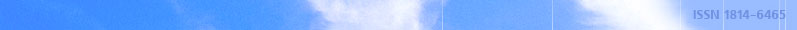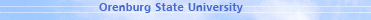december 03, 2021 RU/EN
 Headings of Vestnik Pedagogics Psychology Other

 Search Author Article

# 2012, № 4 (140)

Baklykov A.A., Pushkov S.G. FUZZY LINEAR DYNAMIC SYSTEM AS A SYSTEM OVER SEMIRINGSWe study the problem of describing the dynamics of the process with some degree of uncertainty. The concept of linear dynamical systems over a semiring is introduced, and a number of its properties is described. Fuzzy set is represented as a semimodule over the semiring of real numbers from the interval [0,1]. We study the possibility of such a representation for the theory of fuzzy linear dynamical systems.Key words: linear dynamical systems, semiring, semimodule, fuzzy set, fuzzy systems.

References:

1. Mesarovic M., Takahara Y. General systems theory: mathematical foundations. Moscow: Mir, 1978.

2. Kalman R., Falb P., Arbib M. Topics on mathematical systems theory. Moscow: Mir, 1971.

3. Zadeh L.A. Fuzzy sets // Information and Control, 1965, 8.

4. Averkin A.N., Batyrshin I.Z., Blishun A.F. etc. Fuzzy sets in management models and artificial intelligence. Moscow: Nauka, 1986.

5. Pushkov S.G. On the general theory of fuzzy systems: global states and a fuzzy global response of fuzzy systems // Izvestiya RAN. Teoriya i sistemy upravleniya. — 2001. — №5.

6. Kozhukhar V.A., Pushkov S.G. On the general theory of fuzzy systems: fuzzy linear, fuzzy dynamical systems // Izvestiya RAN. Teoriya i sistemy upravleniya. — 2008. — №5.Editor-in-chief Sergey Aleksandrovich MIROSHNIKOV